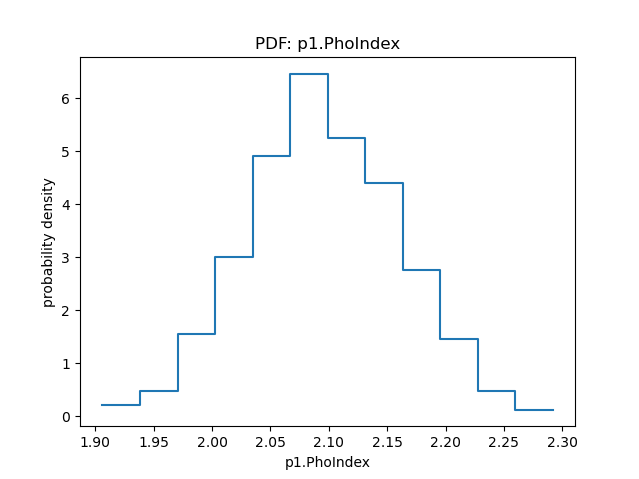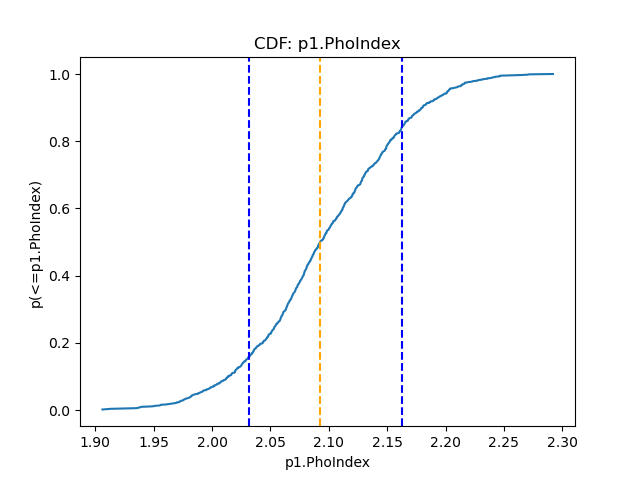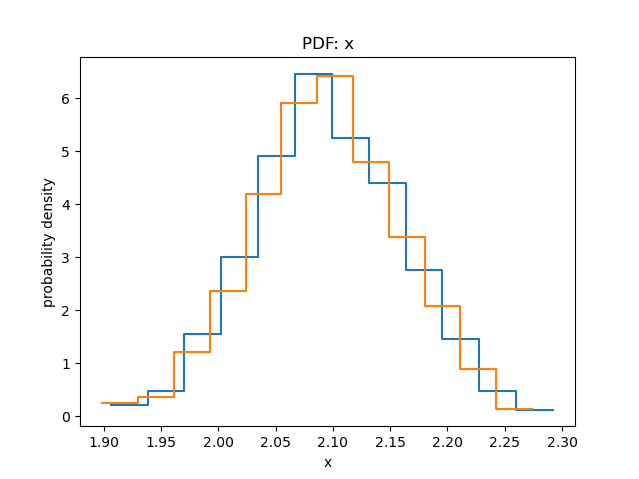# Calculating Model Flux and Flux UncertaintySherpa Threads (CIAO 4.11 Sherpa v1)

## Overview

#### Synopsis:

This thread demonstrates the use of the sample_flux function for calculating the energy flux and associated flux uncertainty due to a Sherpa model, and any model sub-components, previously defined and fit to a data set.

Last Update: 12 Dec 2018 - reviewed for CIAO 4.11, no content change.

## Getting Started

Please follow the Obtaining Data Used in Sherpa thread. In this example, we use the spectral data products for 3C 273, located in the sample_flux sub-directory.

## Fitting a Model to the Spectrum

To begin, we load the spectrum and instrument response files for quasar 3C 273 using the load_pha command, and then fit it with a source model composed of the sum of an absorbed, non-thermal power-law model, and a thermal bremsstrahlung model component. We subtract off the background before fitting, and include only the 0.3-7.0 keV data range in our analysis.

```sherpa> load_pha("3c273.pi")
sherpa> notice(0.3, 7.0)
sherpa> subtract()
sherpa> set_stat("chi2datavar")
sherpa> set_method("simplex")
sherpa> set_model(xsphabs.gal*(xspowerlaw.p1 + xsbremss.kt))
sherpa> gal.nh = 0.07
sherpa> kt.kt = 0.5
sherpa> freeze(gal)
sherpa> fit()
Dataset               = 1
Method                = neldermead
Statistic             = chi2datavar
Initial fit statistic = 1.31027e+11
Final fit statistic   = 36.7109 at function evaluation 1062
Data points           = 44
Degrees of freedom    = 40
Probability [Q-value] = 0.619124
Reduced statistic     = 0.917772
Change in statistic   = 1.31027e+11
p1.PhoIndex    2.10157
p1.norm        0.000215161
kt.kT          0.0530235
kt.norm        0.147625
```

Having performed an initial fit to our data set—choosing χ2 statistics with variance calculated from the data, and the robust Neldermead-Simplex fit optimization—we are ready to run the sample_flux function to obtain an estimate of the energy flux for our source 3C 273, with the corresponding model parameters and associated flux uncertainty.

## Sampling the Model Flux

The sample_flux function takes a number of arguments, the key ones being the model component(s) for which to calculate the associated flux and flux uncertainty, the number of flux simulations to run, and the range of data to consider in the analysis. The returned values include the flux from the full model expression used in the fitting (labeled "original model flux"), and the emission specific to the entered model sub-component(s) (labeled "model component flux").

In this thread example we consider the unabsorbed flux due to the sum of the power-law and bremsstrahlung model components (p1+kt), in the 0.3-7 keV energy range, using only ten simulations (in standard analysis, a larger number, e.g. 100 or more, should be used for a better sampling of the parameter distributions). We also set the Boolean 'correlated' parameter to 'True' to include correlations between the parameters in evaluating the uncertainty.

```sherpa> component = p1 + kt
sherpa> print(component)
(xspowerlaw.p1 + xsbremss.kt)
Param        Type          Value          Min          Max      Units
-----        ----          -----          ---          ---      -----
p1.PhoIndex  thawed      2.10157           -2            9
p1.norm      thawed  0.000215161            0        1e+24
kt.kT        thawed    0.0530235       0.0001          100        keV
kt.norm      thawed     0.147625            0        1e+24
sherpa> sample_flux(component, 0.3, 7 , num=10, correlated=True)
WARNING: hard minimum hit for parameter kt.norm
original model flux = 8.65918e-13, + 2.57331e-14, - 6.47532e-14
model component flux = 1.19932e-12, + 2.37485e-14, - 1.76929e-13

[array([  8.65917552e-13,   8.91650645e-13,   8.01164310e-13]),
array([  1.19932375e-12,   1.22307221e-12,   1.02239524e-12]),
array([[  8.21564859e-13,   2.07850727e+00,   2.11294602e-04,
7.47538782e-02,   0.00000000e+00,   5.21090451e+01],
[  9.13332985e-13,   2.20031856e+00,   2.49522959e-04,
3.15732153e-02,   4.15893823e-01,   4.73587335e+01],
[  8.13005553e-13,   2.08324140e+00,   2.09644233e-04,
6.52457896e-02,   0.00000000e+00,   4.85380112e+01],
[  8.24894984e-13,   2.01949415e+00,   2.03558466e-04,
3.50196475e-02,   4.05637163e-01,   3.73997827e+01],
[  8.01164310e-13,   2.09831690e+00,   2.07992513e-04,
2.96268728e-02,   4.53017743e-01,   4.44595692e+01],
[  8.65917552e-13,   2.11286980e+00,   2.14486800e-04,
5.43212325e-02,   1.01980339e-01,   4.05016021e+01],
[  8.46390566e-13,   2.22125306e+00,   2.27297305e-04,
5.97944580e-02,   2.89697512e-02,   4.18378415e+01],
[  8.91650645e-13,   2.16215494e+00,   2.30460203e-04,
5.61297713e-02,   5.98978317e-02,   0.00000000e+00],
[  9.47833467e-13,   2.01416678e+00,   2.14490894e-04,
5.36037511e-02,   1.92677599e-01,   0.00000000e+00],
[  8.59281447e-13,   2.04371971e+00,   2.16660717e-04,
7.64361525e-02,   0.00000000e+00,   0.00000000e+00],
[  8.59515721e-13,   1.92413815e+00,   2.01195213e-04,
8.77802259e-02,   0.00000000e+00,   0.00000000e+00]])]
```

The screen output shows the 0.3-7 keV energy flux with upper and lower bounds in units of [ergs/s/cm2], for the full model fit to the data, followed by the flux for the specified model component, p1+kt. The flux is obtained as a median of all the flux values in the simulated parameter sets. The default upper and lower bounds are set at 68%, or 1σ, confidence, and may be changed via the 'confidence' parameter.

By default, sample_flux assumes the units of a standard X-ray data set (the 'Xrays' parameter is set to 'True'), returning the energy flux in [ergs/s/cm2]. If 'Xrays' is set to 'False' instead, then the units are determined by the type of input data. For example, for an optical spectrum entered in the units of [W/m2/Hz], defined in frequency (Hz), sample_flux will integrate the spectrum and give the flux in [W/m2].

The sample_flux function also returns a list of arrays. The first two arrays contain the flux with upper and lower bounds for the original model and the specified model component(s), respectively. The last array contains all the simulated parameters with a corresponding flux for the full model expression fit to the data.

To consider another example of the usage of the sample_flux function, we estimate the flux of an individual model component from our full model expression, the thermal bremsstrahlung model component 'kt', within the 0.5-2 keV range at 90% confidence. This time, we choose to use the default 'False' setting for the 'correlated' parameter, increase the number of simulations to 1000, and store the returned values in a variable called sample1.

```sherpa> sample1 = sample_flux(kt, 0.5, 2, num=1000, confidence=90)
WARNING: hard minimum hit for parameter kt.norm
WARNING: Covariance failed for 'kt.norm', trying Confidence...
kt.norm lower bound:	-0.110017
kt.norm +: WARNING: The confidence level lies within (1.800797e+00, 1.805270e+00)
kt.norm upper bound:	1.65541
original model flux = 3.8898e-13, + 6.90776e-14, - 3.92797e-14
model component flux = 3.22165e-15, + 1.257e-13, - 3.2213e-15
sherpa> len(sample1)
3
sherpa> sample1
array([  3.88979928e-13,   4.58057500e-13,   3.49700267e-13])
sherpa> sample1
array([  3.22164809e-15,   1.28921260e-13,   3.48943506e-19])
sherpa> sample1.shape
(1001, 6)
```

The screen output from sample_flux shows the flux values and 90% bounds from both the full model and the single model component 'kt', along with messages from the covariance/confidence calculation of model parameter uncertainties.

The sample1 variable can be used to access the original and model component flux values—sample1 and sample1 respectively—and the flux, parameter values, and statistic for each simulation (sample1). The following returns the fluxes and photon index values:

```sherpa> sample1[:,0]

array([  3.99133964e-13,   4.19290563e-13,   4.01905087e-13,
3.98894185e-13,   3.62579041e-13,   3.88075868e-13,
...
3.78017196e-13,   3.55900075e-13,   3.48047099e-13,
4.01267851e-13,   3.62125869e-13])
sherpa> sample1[:,1]

array([ 2.11911611,  2.01070124,  2.03539575,  2.12093057,  2.10622115,
2.13785499,  2.17173663,  2.04926256,  1.94400878,  2.06317831,
...
2.07720592,  2.05194883,  2.07500349,  2.14064733,  2.03769811,
2.099523  ])
sherpa> print(get_source())
(xsphabs.gal * (xspowerlaw.p1 + xsbremss.kt))
Param        Type          Value          Min          Max      Units
-----        ----          -----          ---          ---      -----
gal.nH       frozen         0.07            0       100000 10^22 atoms / cm^2
p1.PhoIndex  thawed      2.10157           -2            9
p1.norm      thawed  0.000215161            0        1e+24
kt.kT        thawed    0.0530235       0.0001          100        keV
kt.norm      thawed     0.147625            0        1e+24
sherpa> sample1

array([  3.99133964e-13,   2.11911611e+00,   2.23591218e-04,
5.06106843e-02,   1.98395725e-01,   3.73603155e+01])
```

The first element of this array is the flux, and then the thawed parameter values in the same order as listed by the get_source command, with the last element being the statistic value.Changes introduced in CIAO 4.6

The returned value from sample_flux contains the statistic value for each simulation; in the output above, the statistic value is stored in the sample1[:,-1] column.

## Visualizing the Results of the Flux Simulations

We can visualize the distribution of model parameter values resulting from the sample_flux simulations, for a particular model component, using the Sherpa plot_pdf or plot_cdf functions. The plot_pdf function displays a binned probability density function (Figure 1), while plot_cdf shows the cumulative distribution function with lower, median, and upper quantiles (Figure 2).

### Figure 1: Power-law photon index probability density### Figure 1: Power-law photon index probability density

The probablity density for any of the parameters can be displayed using plot_pdf; in this case the p1.PhoIndex parameter:

```sherpa> plot_pdf(sample1[:,1], xlabel="p1.PhoIndex", name="p1.PhoIndex")
```

### Figure 2: Power-law photon index cumulative distribution### Figure 2: Power-law photon index cumulative distribution

The cumulative distribution of the photon index is shown by plot_cdf; the median value is marked by the orange line and 68% quantiles with the blue ones.

```sherpa> plot_cdf(sample1[:,1], xlabel="p1.PhoIndex", name="p1.PhoIndex")
```

## Supplying the Scales for the Simulations

sample_flux assumes a multi-variate Gaussian distribution for the flux simulations, with the Gaussian scales for each parameter given by the covariance matrix (unless covariance fails, in which case confidence is used to calculate the sizes). Correlations are taken into account when the 'correlated' parameter is set to 'True' and the non-diagonal elements from the covariance matrix set the scales.

There is an option to supply the scales to sample_flux for the simulations via the 'scales' parameter, instead of recalculating them with covariance or confidence. If 'correlated=True', the covariance function must be run before sample_flux in order to set the scales. If parameters are uncorrelated ('correlation=False'), a list of scales corresponding to each parameter is needed.

Considering an example using correlated parameters with 'correlated=True', we run the Sherpa covariance command and obtain the resulting covariance matrix to set the scales for the sample_flux simulations.

```sherpa> covar()
WARNING: hard minimum hit for parameter kt.norm
Dataset               = 1
Confidence Method     = covariance
Iterative Fit Method  = None
Fitting Method        = neldermead
Statistic             = chi2datavar
covariance 1-sigma (68.2689%) bounds:
Param            Best-Fit  Lower Bound  Upper Bound
-----            --------  -----------  -----------
p1.PhoIndex       2.10157   -0.0643909    0.0643909
p1.norm       0.000215161 -1.22145e-05  1.22145e-05
kt.kT           0.0530235   -0.0151293    0.0151293
kt.norm          0.147625        -----     0.209062
sherpa> matrix = get_covar_results().extra_output
sherpa> print(matrix)
[[  4.14618861e-03   5.41175266e-07  -2.64643809e-04   3.11705322e-03]
[  5.41175266e-07   1.49194612e-10  -5.35903209e-08   6.36941874e-07]
[ -2.64643809e-04  -5.35903209e-08   2.28895629e-04  -3.09623501e-03]
[  3.11705322e-03   6.36941874e-07  -3.09623501e-03   4.37071172e-02]]
```

The scales for sample_flux may now be defined using the covariance results from get_covar_results, using the command directly, as shown below, or the 'matrix' variable defined above.

```sherpa> sample2 = sample_flux(component, 0.5, 7, num=1000, correlated=True, scales=matrix)
original model flux = 7.58064e-13, + 3.56314e-14, - 3.02093e-14
model component flux = 8.60746e-13, + 3.99164e-14, - 3.37391e-14

sherpa> print(sample2[0:20,1])
[ 2.13118009  2.03690006  2.09549963  2.06459314  2.06429081  2.18076098
2.09183984  2.15280255  1.97965206  2.06891094  2.13570307  2.11845219
2.11673748  2.10563833  2.18444915  2.15003379  2.0844819   2.09831156
1.99372799  2.13450436]
```

### Figure 3: Comparison of the power-law distributions### Figure 3: Comparison of the power-law distributions

Here we compare the power-law distribution from Figure 1 to that obtained when using the covariance matrix (the red dotted line):

```sherpa> plot_pdf(sample1[:,1])
sherpa> plot_pdf(sample2[:,1], overplot=True)
sherpa> set_histogram(['*.color', 'red', 'line.style', 'shortdash'])
```

## Saving the Simulation Results

The arrays of model parameter values and associated fluxes from our two sample_flux runs may be written to file using the Sherpa save_arrays command, as shown below. Here, we write the power-law photon index and thermal bremsstrahlung temperature arrays from the first sample_flux run to the ASCII file sample1.dat, specifying data column names and overwrite the existing sample1.dat file.

```sherpa> save_arrays("sample1.dat", [sample1[:,1], sample1[:,3]], ['Photon Index', 'Temperature'], clobber=True)
```

## Scripting It

The file, fit.py , performs the primary commands used above. It can be executed by typing exec(open("flux_script.py").read()) on the Sherpa command line. The Sherpa script command may be used to save everything typed on the command line in a Sherpa session:

```sherpa> script(filename="sherpa.log", clobber=False)
```

(Note that restoring a Sherpa session from such a file could be problematic since it may include syntax errors, unwanted fitting trials, et cetera.)

## History

 13 Dec 2012 original version 10 Dec 2013 Updated for CIAO 4.6: the return value from sample_flux now includes the statistic value of each simulation. 18 Mar 2015 Updated for CIAO 4.7, no content change. 12 Jul 2018 reviewed for CIAO 4.10, no content change. 12 Dec 2018 reviewed for CIAO 4.11, no content change.